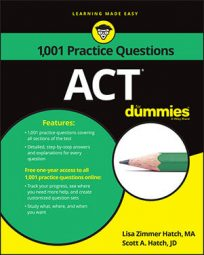##### ACTThere's no getting around it: you'll probably have to solve some questions on the ACT Math exam that deal with circles. To solve the following practice questions, you'll need to know the formulas for the area of a circle and the general equation for a circle.

## Practice questions

1. The figure shows a portion of the graph of y = (1.5)x and a circle with center (3, 1).

The two meet at the point indicated in the graph. Which is nearest to the area of the circle in square units?

2. Which of the following represents the equation of a circle in the standard xy-coordinate plane that is tangent to the x-axis at 3 units and to the y-axis at 3 units?

A. x2 + y2 = 9 B. (x + 3)2 + (y – 3)2 = 9 C. (x – 3)2 + (y – 3)2 = 9 D. (x – 3)2 + (y – 3)2 = 6 E. (x + 3)2 – (y + 3)2 = 6

1. The correct answer is Choice (A). Don't let the equation with the x exponent throw you. Just use a couple of familiar formulas.

The formula for the area of a circle is

So find the radius of the circle, apply it to the formula, and you're done.

The radius is the distance from (3, 1) to the point on the circle that intersects with the graph. Find the coordinates of that point, and you can use the handy dandy distance formula to discover the length of the radius.

The point's x-coordinate is obvious. The dotted line on the figure indicates that it's 2. The y-coordinate, then, is what you get when you plug 2 in for x in the equation of the curve:

The y-coordinate is 2.25. So the coordinates of the second point are (2, 2.25).

With the coordinates of the two points, you can use the distance formula to find the radius.

The radius of the circle is

Plug that value into the area formula (Don't bother to find the square root of 2.5625 because you just square it again in the area formula):

2. The correct answer is Choice (C). For this problem, you need to know the general equation for a circle: (xh)2 + (yk)2 =r2, where h and k are the x- and y-coordinates of the center of the circle and r is its radius.

Because the circle is tangent to the x and y at 3 units, the radius of the circle is 3. Eliminate Choices (D) and (E) because they don't have 32 on the right side of the equation.

Choice (A) is wrong because it's the equation for a circle with a center on the origin (0, 0). There's no way this circle's center is on the origin if it's touching both axes.

Choice (B) adds rather than subtracts within the first parentheses, which would be true only if the center were (–3, 3).

Choice (C) is the only equation in proper format for a circle with a center point of (3, 3).